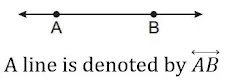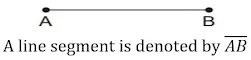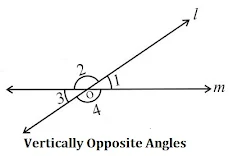# Chapter 5 Lines and Angles Class 7 Notes Maths

Chapter 5 Lines and Angles Class 7 Notes Maths is available on this page that will be make sure that a student has understood the specifics of every chapter in clear and precise manner. Class 7 Maths Revision Notes will help the students to recall information with more precision and faster. NCERT Notes becomes a vital resource for all the students to self-study from NCERT textbooks carefully. You will find NCERT Solutions for Class 7 Chapter 5 Maths through which you will be able to solve the difficult problems in a given in exercise.Recall

• A Point means a location.

• A line is a straight that extends endlessly in both directions.• A line segment is the part of a line between two points.• A ray is part of a line that starts at one point and extends endlessly in another direction.• An angle is formed when lines or line segments meet.Related Angles

• When the sum of the measures of two angles is 90°, the angles are called complementary angles.

• Whenever two angles are complementary, each angle is said to be the complement of the other angle.• When the sum of the measures of two angles is 180°, the angles are called Supplementary angles.

• When two angles are supplementary, each angle is said to be the supplement of the other.• Two angles with a common vertex and common arm but no common interior points are called Adjacent angles.

• These angles are such that:
(i) they have a common vertex;
(ii) they have a common arm; and
(iii) the non-common arms are on either side of the common arm.

• A linear pair is a pair of adjacent angles whose non-common sides are opposite rays.

• Vertically Opposite Angles: Two angles formed by two intersecting lines having no common arm. When two lines intersect, the vertically opposite angles so formed are equal.Pair of Lines

• Intersecting Lines: Two or more lines that have one and only one point in common. The common point where all the intersecting lines meet is called the point of intersection.

• A line that intersects two or more lines at distinct points is called a transversal.

• Parallel lines: Two lines in the same plane that are at equal distance from each other and never meet.

• Alternate interior angle : The pair of angles on opposite sides of the transversal but inside the two lines are called alternate interior angles.

• Alternate exterior angle : The pair of angles on the opposite sides of the transversal but outside the two lines
are called alternate exterior angles

• When two lines are intersected by a transversal, eight angles are formed.Interior angles: ∠3, ∠4, ∠5, ∠6
Exterior angles: ∠1, ∠2, ∠7, ∠8
Pairs of corresponding angles: ∠1 and ∠5, ∠2 and ∠6, ∠3 and ∠7, ∠4 and ∠8
Pairs of Alternate interior angles: ∠3 and ∠6, ∠4 and ∠5
Pairs of Alternate exterior angles: ∠1 and ∠8, ∠2 and ∠7
Pairs of interior angles on the same side of the transversal: ∠3 and ∠5, ∠4 and ∠6.

Transversal of parallel lines

• If two parallel lines are intersected by a transversal, each pair of:
(i) corresponding angles are congruent
(ii) alternate interior angles are congruent
(iii) alternate exterior angles are congruent
(iv) interior angles on the same side of the transversal are supplementary.